login  home  contents  what's new  discussion  bug reports help  links  subscribe  changes  refresh  edit

## Sedenion Algebra is Frobenius in just one way!

Linear operators over a 16-dimensional vector space representing Sedenion Algebra

Ref:

We need the Axiom LinearOperator library.

fricas
)library CARTEN MONAL PROP LOP CALEY
CartesianTensor is now explicitly exposed in frame initial
CartesianTensor will be automatically loaded when needed from
/var/aw/var/LatexWiki/CARTEN.NRLIB/CARTEN
Monoidal is now explicitly exposed in frame initial
Monoidal will be automatically loaded when needed from
/var/aw/var/LatexWiki/MONAL.NRLIB/MONAL
Prop is now explicitly exposed in frame initial
Prop will be automatically loaded when needed from
/var/aw/var/LatexWiki/PROP.NRLIB/PROP
LinearOperator is now explicitly exposed in frame initial
LinearOperator will be automatically loaded when needed from
/var/aw/var/LatexWiki/LOP.NRLIB/LOP
CaleyDickson is now explicitly exposed in frame initial
CaleyDickson will be automatically loaded when needed from
/var/aw/var/LatexWiki/CALEY.NRLIB/CALEY

Use the following macros for convenient notation

fricas
-- summation
macro Σ(x,i,n)==reduce(+,[x for i in n])
Type: Void
fricas
-- subscript
macro sb == subscript
Type: Void

ℒ is the domain of 16-dimensional linear operators over the rational functions ℚ (Expression Integer), i.e. ratio of polynomials with integer coefficients.

fricas
dim:=16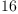(1)
Type: PositiveInteger?
fricas
macro ℂ == CaleyDickson
Type: Void
fricas
macro ℚ == Expression Integer
Type: Void
fricas
ℒ := LinearOperator(OVAR ['0,'1,'2,'3,'4,'5,'6,'7,'8,'9,'10,'11,'12,'13,'14,'15], ℚ)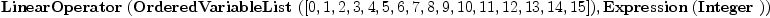(2)
Type: Type
fricas
ⅇ:List ℒ      := basisOut()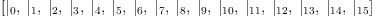(3)
Type: List(LinearOperator(OrderedVariableList([0,1,2,3,4,5,6,7,8,9,10,11,12,13,14,15]),Expression(Integer)))
fricas
ⅆ:List ℒ      := basisIn()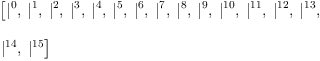(4)
Type: List(LinearOperator(OrderedVariableList([0,1,2,3,4,5,6,7,8,9,10,11,12,13,14,15]),Expression(Integer)))
fricas
I:ℒ:=;   -- identity for composition
Type: LinearOperator(OrderedVariableList([0,1,2,3,4,5,6,7,8,9,10,11,12,13,14,15]),Expression(Integer))
fricas
X:ℒ:=[2,1]; -- twist
Type: LinearOperator(OrderedVariableList([0,1,2,3,4,5,6,7,8,9,10,11,12,13,14,15]),Expression(Integer))

Now generate structure constants for Sedenion Algebra

The basis consists of the real and imaginary units. We use quaternion multiplication to form the "multiplication table" as a matrix. Then the structure constants can be obtained by dividing each matrix entry by the list of basis vectors.

Split-complex, co-quaternions, split-octonions and seneions can be specified by Caley-Dickson parameters

fricas
--q0:=sb('q,)
q0:=1  -- not split-complex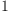(5)
Type: PositiveInteger?
fricas
--q1:=sb('q,)
q1:=1  -- not co-quaternion(6)
Type: PositiveInteger?
fricas
--q2:=sb('q,)
q2:=1  -- not split-octonion(7)
Type: PositiveInteger?
fricas
--q3:=sb('q,)
q3:=1  -- not split-sedennion(8)
Type: PositiveInteger?
fricas
QQ := ℂ(ℂ(ℂ(ℂ(ℚ,'i,q0),'j,q1),'k,q2),'l,q3);
Type: Type

Basis: Each B.i is a sedennion number

fricas
B:List QQ := map(x +-> hyper x,1$SQMATRIX(dim,ℚ)::List List ℚ)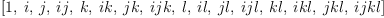(9) Type: List(CaleyDickson(CaleyDickson(CaleyDickson(CaleyDickson(Expression(Integer),i,1),j,1),k,1),l,1)) fricas -- Multiplication table: M:Matrix QQ := matrix [[B.i*B.j for i in 1..dim] for j in 1..dim]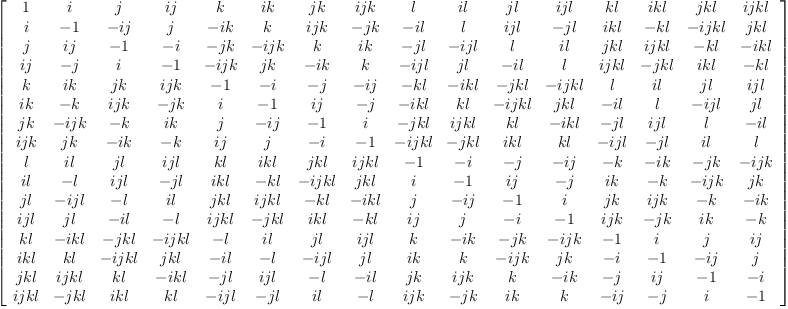(10) Type: Matrix(CaleyDickson(CaleyDickson(CaleyDickson(CaleyDickson(Expression(Integer),i,1),j,1),k,1),l,1)) fricas -- Function to divide the matrix entries by a basis element S(y) == map(x +-> real real real real(x/y),M) Type: Void fricas -- The result is a nested list ѕ :=map(S,B)::List List List ℚ; fricas Compiling function S with type CaleyDickson(CaleyDickson( CaleyDickson(CaleyDickson(Expression(Integer),i,1),j,1),k,1),l,1) -> Matrix(Expression(Integer)) Type: List(List(List(Expression(Integer)))) fricas -- structure constants form a tensor operator Y := Σ(Σ(Σ(ѕ(i)(k)(j)*ⅇ.i*ⅆ.j*ⅆ.k, i,1..dim), j,1..dim), k,1..dim); Type: LinearOperator(OrderedVariableList([0,1,2,3,4,5,6,7,8,9,10,11,12,13,14,15]),Expression(Integer)) fricas arity Y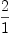(11) Type: Prop(LinearOperator(OrderedVariableList([0,1,2,3,4,5,6,7,8,9,10,11,12,13,14,15]),Expression(Integer))) fricas matrix [[(ⅇ.i*ⅇ.j)/Y for i in 1..dim] for j in 1..dim]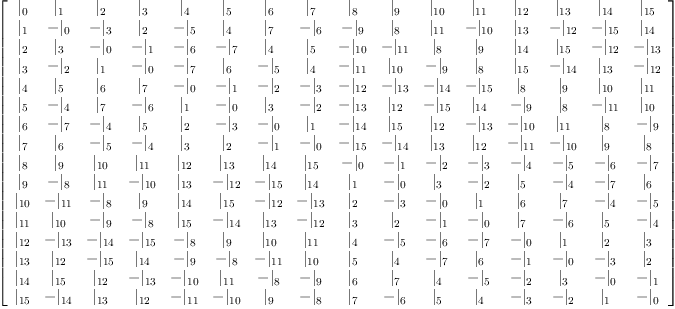(12) Type: Matrix(LinearOperator(OrderedVariableList([0,1,2,3,4,5,6,7,8,9,10,11,12,13,14,15]),Expression(Integer))) A scalar product is denoted by the (2,0)-tensor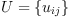fricas U:=Σ(Σ(script('u,[[],[i,j]])*ⅆ.i*ⅆ.j, i,1..dim), j,1..dim); Type: LinearOperator(OrderedVariableList([0,1,2,3,4,5,6,7,8,9,10,11,12,13,14,15]),Expression(Integer)) ## Definition 1 We say that the scalar product is associative if the tensor equation holds:  Y = Y U U  In other words, if the (3,0)-tensor: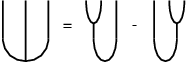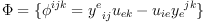(13) (three-point function) is zero. Using the LinearOperator domain in Axiom and some carefully chosen symbols we can easily enter expressions that are both readable and interpreted by Axiom as "graphical calculus" diagrams describing complex products and compositions of linear operators. fricas ω:ℒ :=(Y*I)/U - (I*Y)/U; Type: LinearOperator(OrderedVariableList([0,1,2,3,4,5,6,7,8,9,10,11,12,13,14,15]),Expression(Integer)) ## Definition 2 An algebra with a non-degenerate associative scalar product is called a [Frobenius Algebra]?. We may consider the problem where multiplication Y is given, and look for all associative scalar products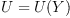This problem can be solved using linear algebra. fricas )expose MCALCFN MultiVariableCalculusFunctions is now explicitly exposed in frame initial J := jacobian(ravel ω,concat map(variables,ravel U)::List Symbol); Type: Matrix(Expression(Integer)) fricas --u := transpose matrix [concat map(variables,ravel U)::List Symbol]; --J::OutputForm * u::OutputForm = 0 nrows(J),ncols(J)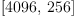(14) Type: Tuple(PositiveInteger?) The matrix J transforms the coefficients of the tensor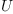into coefficients of the tensor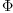. We are looking for the general linear family of tensors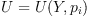such that J transformsinto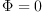for any such. If the null space of the J matrix is not empty we can use the basis to find all non-trivial solutions for U: fricas Ñ:=nullSpace(J); Type: List(Vector(Expression(Integer))) fricas ℰ:=map((x,y)+->x=y, concat map(variables,ravel U), entries Σ(sb('p,[i])*Ñ.i, i,1..#Ñ) )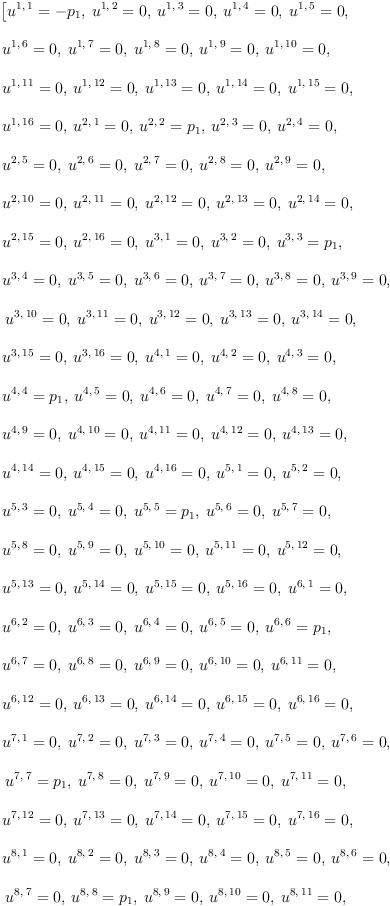(15) Type: List(Equation(Expression(Integer))) This defines a family of Frobenius algebras: fricas zero? eval(ω,ℰ)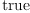(16) Type: Boolean The pairing is necessarily diagonal! fricas Ų:ℒ := eval(U,ℰ)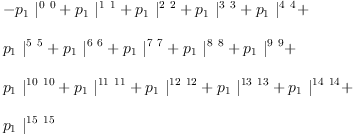(17) Type: LinearOperator(OrderedVariableList([0,1,2,3,4,5,6,7,8,9,10,11,12,13,14,15]),Expression(Integer)) fricas matrix [[(ⅇ.i ⅇ.j)/Ų for i in 1..dim] for j in 1..dim]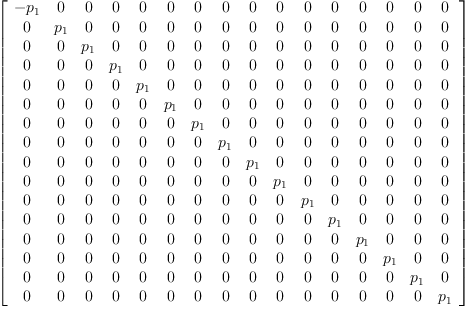(18) Type: Matrix(LinearOperator(OrderedVariableList([0,1,2,3,4,5,6,7,8,9,10,11,12,13,14,15]),Expression(Integer))) The scalar product must be non-degenerate: fricas Ů:=determinant [[retract((ⅇ.i * ⅇ.j)/Ų) for j in 1..dim] for i in 1..dim]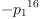(19) Type: Expression(Integer) fricas factor Ů(20) Type: Factored(Expression(Integer)) ## Definition 3 Co-pairing Solve the Snake Relation as a system of linear equations. fricas Um:=matrix [[(ⅇ.i*ⅇ.j)/Ų for i in 1..dim] for j in 1..dim]; Type: Matrix(LinearOperator(OrderedVariableList([0,1,2,3,4,5,6,7,8,9,10,11,12,13,14,15]),Expression(Integer))) fricas mU:=transpose inverse map(retract,Um); Type: Matrix(Expression(Integer)) fricas Ω:=Σ(Σ(mU(i,j)*(ⅇ.i*ⅇ.j), i,1..dim), j,1..dim)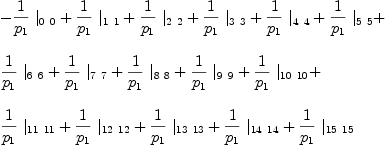(21) Type: LinearOperator(OrderedVariableList([0,1,2,3,4,5,6,7,8,9,10,11,12,13,14,15]),Expression(Integer)) fricas matrix [[Ω/(ⅆ.i*ⅆ.j) for i in 1..dim] for j in 1..dim]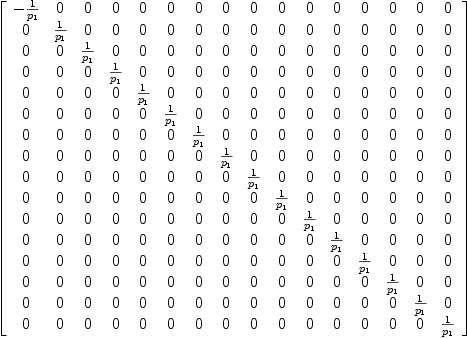(22) Type: Matrix(LinearOperator(OrderedVariableList([0,1,2,3,4,5,6,7,8,9,10,11,12,13,14,15]),Expression(Integer))) Check "dimension" and the snake relations. fricas d:ℒ:= Ω / X / Ų(23) Type: LinearOperator(OrderedVariableList([0,1,2,3,4,5,6,7,8,9,10,11,12,13,14,15]),Expression(Integer)) fricas test ( I Ω ) / ( Ų I ) = I(24) Type: Boolean fricas test ( Ω I ) / ( I Ų ) = I(25) Type: Boolean ## Definition 4 Co-algebra Compute the "three-point" function and use it to define co-multiplication. Too slow:  \begin{axiom} W:=(Y,I)/Ų; λ:=(Ω,I,Ω)/(I,W,I) \end{axiom}  fricas λ:= (I,Ω) / (Y,I)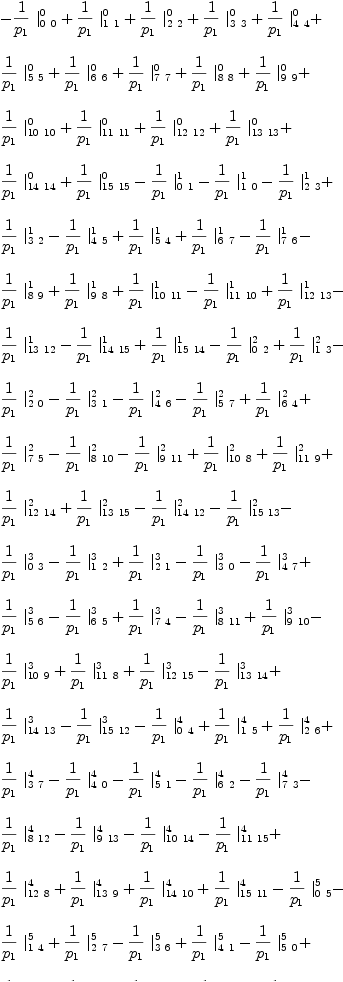(26) Type: LinearOperator(OrderedVariableList([0,1,2,3,4,5,6,7,8,9,10,11,12,13,14,15]),Expression(Integer)) fricas test( (Ω,I) / (I,Y) = λ )(27) Type: Boolean ## Frobenius Condition Like Octonion algebra Sedenion algebra also fails the Frobenius Condition! Too slow to complete here:  \begin{axiom} Χ := Y / λ ; Χr := (λ,I)/(I,Y) test(Χr = Χ ) Χl := (I,λ)/(Y,I); --test( Χl = Χ ) test( Χr = Χl ) \end{axiom}  Perhaps this is not too surprising since like Octonion Seden algebra is non-associative (in fact also non-alternative). Nevertheless Sedenions are "Frobenius" in a more general sense just because there is a non-degenerate associative pairing. i = Unit of the algebra fricas i:=ⅇ.1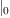(28) Type: LinearOperator(OrderedVariableList([0,1,2,3,4,5,6,7,8,9,10,11,12,13,14,15]),Expression(Integer)) fricas test i / λ = Ω(29) Type: Boolean Handle fricas H:ℒ := λ / X / Y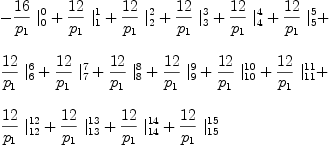(30) Type: LinearOperator(OrderedVariableList([0,1,2,3,4,5,6,7,8,9,10,11,12,13,14,15]),Expression(Integer)) ## Definition 5 Co-unit  i U  fricas ι:ℒ:= ( i I ) / ( Ų )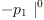(31) Type: LinearOperator(OrderedVariableList([0,1,2,3,4,5,6,7,8,9,10,11,12,13,14,15]),Expression(Integer)) Y=U ι  fricas test Y / ι = Ų(32) Type: Boolean For example: fricas ex1:=[q=1,p=1]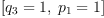(33) Type: List(Equation(Polynomial(Integer))) fricas Ų0:ℒ :=eval(Ų,ex1)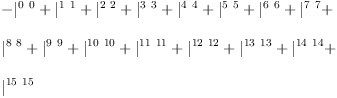(34) Type: LinearOperator(OrderedVariableList([0,1,2,3,4,5,6,7,8,9,10,11,12,13,14,15]),Expression(Integer)) fricas Ω0:ℒ :=eval(Ω,ex1)$ℒ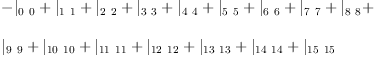(35)
Type: LinearOperator(OrderedVariableList([0,1,2,3,4,5,6,7,8,9,10,11,12,13,14,15]),Expression(Integer))
fricas
λ0:ℒ  :=eval(λ,ex1)$ℒ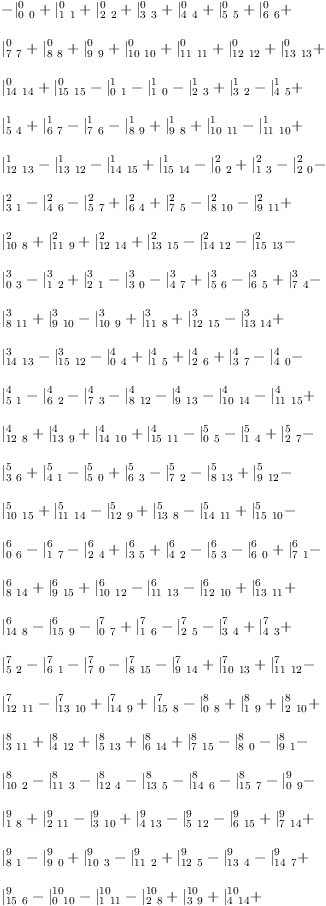(36) Type: LinearOperator(OrderedVariableList([0,1,2,3,4,5,6,7,8,9,10,11,12,13,14,15]),Expression(Integer)) fricas H0:ℒ :=eval(H,ex1)$ℒ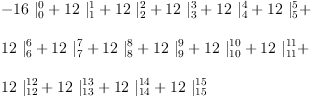(37)
Type: LinearOperator(OrderedVariableList([0,1,2,3,4,5,6,7,8,9,10,11,12,13,14,15]),Expression(Integer))

 Subject:   Be Bold !! ( 14 subscribers )